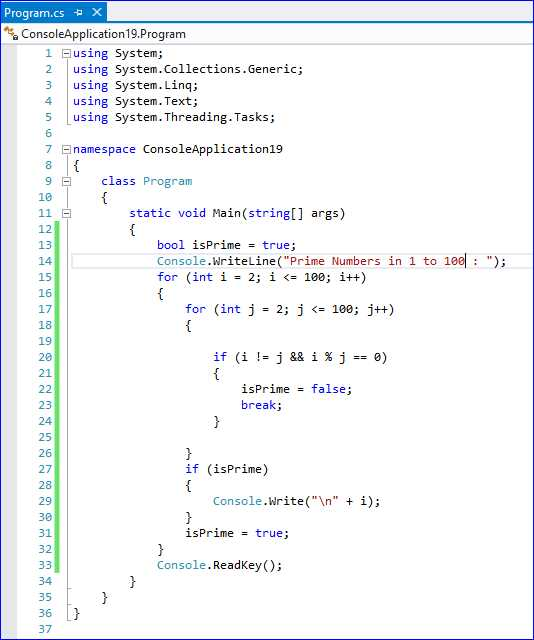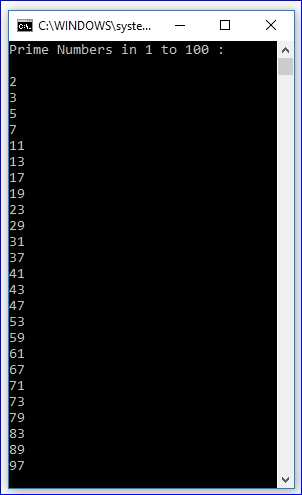# How can Display All the Prime Numbers Between 1 to 100 ?

Posted by  Gurmeet Kaur
684  View(s)
Ratings:
Rate this:

Display All the Prime Numbers Between 1 to 100.

1.##### Re: How can Display All the Prime Numbers Between 1 to 100 ?

Prime Number between 1 to 100

Here I am writing a program in C # which searches for the prime number, a major number is more than 1 natural number, which has 1 and there is no positive separator other than itself.

Like : 3,5,7 etc.

```using System;
using System.Collections.Generic;
using System.Linq;
using System.Text;

namespace ConsoleApplication19
{
class Program
{
static void Main(string[] args)
{
bool isPrime = true;
Console.WriteLine("Prime Numbers in 1 to 100 : ");
for (int i = 2; i <= 100; i++)
{
for (int j = 2; j <= 100; j++)
{

if (i != j && i % j == 0)
{
isPrime = false;
break;
}

}
if (isPrime)
{
Console.Write("\n" + i);
}
isPrime = true;
}